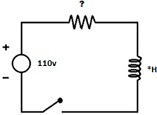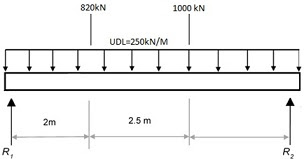# Demonstrate how different models of engineering systems using first-order differential equations can be used to solve engineering problems

Learning Outcome 1: Review models of engineering systems using ordinary differential equations

Learning Outcome 2: Formulate and solve first order differential equations related to engineering systems

Learning Outcome 3: Formulate and solve second order homogeneous and non-homogeneous differential equations related to engineering systems

Learning Outcome 4: Calculate solutions to linear ordinary differential equations using Laplace transforms

Learning Outcome 5: Demonstrate how different models of engineering systems using first-order differential equations can be used to solve engineering problems

Learning Outcome 6: Critically evaluate first and second-order differential equations when generating the solutions to engineering situations using models of engineering systems

For the following questions, include any assumptions made and show all working out.

Question 1) An object falls from rest from the top of the Burj Khalifa building in Dubai (height +*) and accelerates under the force of gravity. Construct and solve a differential equation to determine:
a) How long, in seconds, it would take the unobstructed object to reach the ground?
b) How far from the ground would the object be after 8 seconds?
Give answers correct to 2 decimal places.

Question 2) A system has a constant force of 110V applied and *H of inductance. If it takes 1 second for the system`s current to reach *A upon closing a switch (so that the current at t = 0 is 0A), using a calculator or approximation, what would the resistance be? Give an exact figure for your answer. Do not round up or down.Question 3) A tank of hot liquid at 300+* °C will cool to 200+*°C in one hour if it is surrounded by an environment of 10+*°C . Suppose a heater is placed in the tank and the heater itself is a constant temperature of 305+*°C . What does the cooling coefficient from the heater to the liquid need to be in order for the temperature of the liquid in the tank to stay constant?

Question 4) Use Laplace Transforms to solve the following Ordinary Differential Equation:

D3x - D2x=0

given that the initial conditions are:

x(0)=x` (0)=x`` (0)=*

Question 5) Using Laplace Transforms and partial fractions, solve the following Ordinary Differential Equation:

dx/dt-x=2sin(t)

given the initial condition:

x(0)=0

Question 6) The company you work for produces high quality produce for the food industry. One of the automated food mixing vessels as shown below is to be used in the production of a new sauce.

Each vessel contains 2000 litres of sauce recipe which is pumped out of the vessel to be bottled at a rate of 60 litres/hr. Preservatives are added to the mixture at a rate of 80 litres/hr to which 8g of protected secret ingredient are added per litre of mix. From a team meeting you have been asked to confirm the equation below that the manufacture have stated by proving how it was formulated-

dx/dt = 640-6x/(200+2t)

Also confirm the amount of secret ingredient that is present in the vessel after 3 hours of production and how this is impacted by adding 10g of secret ingredient.Further to this critically evaluate an aspect of the model that could be developed as a refinement.

Question 7) The latest project you are involved in is designing the load bearing support structure for a new mezzanine floor to be constructed in the design department. The potential design shown below requires various calculations to be made to ensure it has a factor of safety involved.Modeland solve the 2nd order differential equation to clarify the slope and deflection occurring at the centre point of the 7m long *beam based on the exaggerated loadings (shown above) that have been proposed to test the beam to a maximum level.Further to this critically evaluate an aspect of the model that could be developed as an improvement.